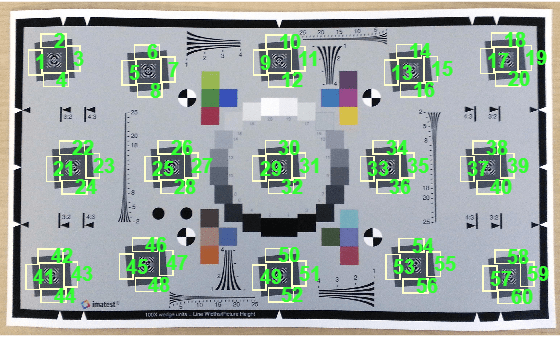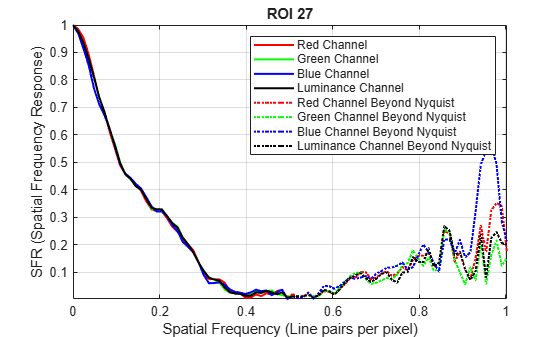# plotSFR

Plot spatial frequency response of edge

## Syntax

``plotSFR(sharpnessMeasurementTable)``
``plotSFR(sharpnessMeasurementTable,Name,Value)``

## Description

````plotSFR(sharpnessMeasurementTable)` plots the spatial frequency response (SFR) in a sharpness measurement table or aggregate sharpness measurement table.```

example

````plotSFR(sharpnessMeasurementTable,Name,Value)` plots the SFR, specifying additional parameters to control aspects of the display.```

## Examples

collapse all

This example shows how to display the spatial frequency response (SFR) plot of a specified subset of the 60 slanted edge ROIs on an Imatest® eSFR chart.

Read an image of an eSFR chart into the workspace.

`I = imread("eSFRTestImage.jpg");`

Create an `esfrChart` object, then display the chart with ROI annotations. The 60 slanted edge ROIs are labeled with green numbers.

```chart = esfrChart(I); displayChart(chart,displayGrayROIs=false,... displayColorROIs=false,displayRegistrationPoints=false)```Measure the edge sharpness in all ROIs and return the measurements in `sharpnessTable`.

`sharpnessTable = measureSharpness(chart);`

Display the SFR plot of ROIs 26 and 27 only.

`plotSFR(sharpnessTable,ROIIndex=[26 27]);`## Input Arguments

collapse all

SFR measurements of edges, specified as a sharpness table or aggregate sharpness table with m rows:

• When `sharpnessMeasurementTable` is a sharpness table, m is the number of sampled ROIs.

• When `sharpnessMeasurementTable` is an aggregate sharpness table, m is either 1 or 2, corresponding to the number of sampled orientations.

To obtain a sharpness table or aggregate sharpness table, use the `measureSharpness` function.

Data Types: `table`

### Name-Value Arguments

Specify optional pairs of arguments as `Name1=Value1,...,NameN=ValueN`, where `Name` is the argument name and `Value` is the corresponding value. Name-value arguments must appear after other arguments, but the order of the pairs does not matter.

Before R2021a, use commas to separate each name and value, and enclose `Name` in quotes.

Example: `plotSFR(myTable,'ROIIndex',2)` displays the measured sharpness only of ROI 2.

ROI indices to display, specified as the comma-separated pair consisting of `'ROIIndex'` and a scalar or vector of integers in the range [1, 60]. The indices match the ROI numbers displayed by `displayChart`.

• When `sharpnessMeasurementTable` is a sharpness table, by default `plotSFR` creates only one figure, showing the SFR plot from the first row of the table.

• When `sharpnessMeasurementTable` is an aggregate sharpness table, `plotSFR` ignores the specified `ROIIndex`, and creates one figure for each row in the table.

Example: `29:32`

Data Types: `single` | `double` | `int8` | `int16` | `int32` | `uint8` | `uint16` | `uint32`

Display plot legend, specified as the comma-separated pair consisting of `'displayLegend'` and `true` or `false`. When `displayLegend` is `true`, the SFR plot shows a legend that identifies the different curves on the plot.

Data Types: `logical`

Display plot title, specified as the comma-separated pair consisting of `'displayTitle'` and `true` or `false`. When `displayTitle` is `true`, the SFR plot shows a title that indicates the individual ROI index or aggregate ROI orientation.

Data Types: `logical`

Axes handle of the displayed image object, specified as the comma-separated pair consisting of `'Parent'` and an axes handle. `Parent` specifies the parent of the image object created by `plotSFR`.

## Version History

Introduced in R2017b